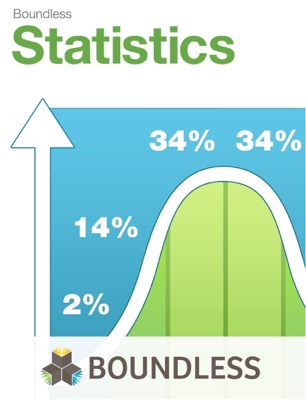### Statistics - Boundless

Relase Date: August 01, 2013
Publisher: Boundless
Category: Statistics

# Summary

Introduction to Statistics is a college-level, introductory textbook that covers the fascinating subject of Statistics, a branch of mathematics highly relevant to everyday situations. Boundless works with subject matter experts to select the best open educational resources available on the web, review the content for quality, and create introductory, college-level textbooks designed to meet the study needs of university students. This textbook covers: Introduction to Statistics and Statistical Thinking â€“ Overview   Statistics in Practice -- Observational Studies, Controlled Experiments   Visualizing Data -- The Histogram, Graphing Data   Frequency Distributions -- Frequency Distributions for Quantitative Data, Frequency Distributions for Qualitative Data   Describing, Exploring, and Comparing Data -- Central Tendancy, Measures of Relative Standing, The Law of Averages, Further Considerations for Data   Measures of Variation -- Describing Variability   Sampling -- Populations and Samples, Sample Surveys, Sampling Distributions, Errors in Sampling, Sampling Examples   Probability -- What Are the Chances?, Probability Rules, More About Chance   Probability and Variability -- Discrete Random Variables, The Binomial Random Variable, Other Random Variables   Continuous Random Variables -- The Normal Curve, Normal Approximation for Data, Measurement Error, Expected Value and Standard Error, Normal Approximation for Probability Histograms   Correlation and Regression -- Correlation, More About Correlation, Regression, The Regression Line, R.M.S. Error for Regression, Multiple Regression   Estimation and Hypothesis Testing -- Estimation, Confidence Intervals, Hypothesis Testing, Hypothesis Testing with Two Samples, Comparing More than Two Means, The F-Test, Analysis of Variance (Repeated Measures)   Other Hypothesis Tests -- The T-Test, The Chi-Squared Test, Tests for Ranked Data, Nonparametric Statistics   A Closer Look at Tests of Significance -- Which Test?, A Closer Look at Tests of Significance